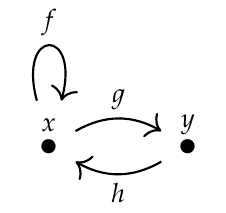>>**Puzzle 104.** How many paths of length \\(n\\) go from \\(x\\) to \\(x\\) in this graph?

>The answer is a famous sequence of numbers. So, you're getting a famous sequence from the free category on a graph!

When \\(n=0\\),
\\[\lbrace id_x \rbrace, \\\\
1,
\\]
when \\(n=1\\),
\\[\lbrace f \rbrace, \\\\
1,
\\]
when \\(n=2\\),
\\[\lbrace f\circ f, g \circ h \rbrace, \\\\
2,
\\]
when \\(n=3\\),
\\[\lbrace f\circ f\circ f, f\circ g \circ h, g\circ h\circ f \rbrace, \\\\
3,
\\]
when \\(n=4\\),
\\[\lbrace
f\circ f\circ f\circ f,
f\circ f\circ g \circ h,
f\circ g \circ h\circ f,
g\circ h\circ f \circ f,
g\circ h\circ g \circ h
\rbrace, \\\\
5,\\\\
\\]
when \\(n=5\\),
\\[\lbrace
f\circ f\circ f\circ f\circ f,
f\circ f\circ f\circ g \circ h,
f\circ f\circ g\circ h\circ f,
f\circ g\circ h\circ f \circ f, \\\\
g\circ h\circ f\circ f\circ f,
g\circ h\circ g\circ h\circ f,
g\circ h\circ f\circ g\circ h,
f\circ g\circ h\circ g \circ h
\rbrace, \\\\
8,\\\\
\cdots
\\]

It's the old Fibonacci sequence.